# How can I solve this equation

## Solve equations (various types) explanation

Here you will learn what different types of equations there are and how to solve them. Let's look at this:

• A Explanation what types of equations there are.
• Examples to recognize the type of equation.
• Tasks / exercises so that you can practice this yourself.
• A Video for solving equations.
• A Question and answer area to this topic.

Tip: We'll look at different types of equations here, with examples to help them. If available, you will find the links to the article under the respective examples, where the solution is discussed.

### Explanation Solve equation

In order to be able to solve an equation, one must first recognize what kind of equation it is. Let's start with simple equations and then move on to more sophisticated equations, systems of equations and inequalities.

Simple equations:

Very simple equations have signs for addition (+) and subtraction (-). These look like this, for example: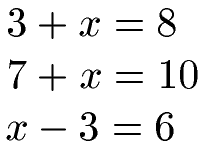Such simple equations can be solved with additions and subtractions. You can learn how to do this under Solving / rearranging equations.

Linear equations:

Linear equations are also relatively simple equations. The highest exponent of the variable is 1, i.e. x1 is not exceeded. Linear equations take the form of the next equation or can be expressed in this form: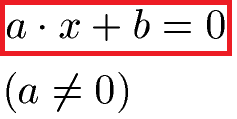Some examples of linear equations: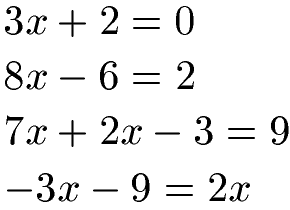Linear equations can be solved by addition, subtraction, multiplication and division. You can learn how this works by solving linear equations.

For a linear function, the power was limited to 1, for example 3x1. In a quadratic equation (or quadratic function) a 2 occurs as the exponent of the variable. The general form of a quadratic equation looks like this: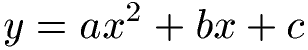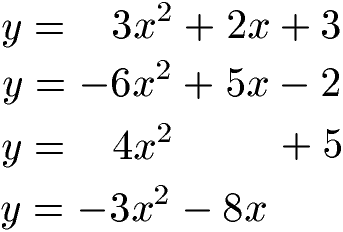Quadratic equations can be solved, for example, with the PQ formula or the midnight formula (ABC formula). You can learn how to do this under Solve a quadratic equation / function.

Cubic equations:

With the variable to a power of 1, we have a linear equation. A quadratic equation has the highest exponent 2. If the exponent for the variable is 3, for example 6x3, then it is a cubic equation. The general form looks like this: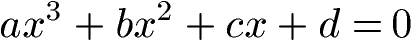Examples of cubic equations: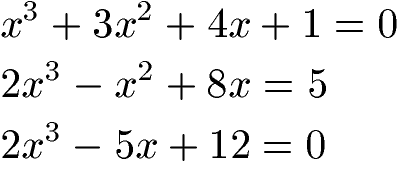There are several ways to solve cubic equations. One of them is the polynomial division. You will learn this under polynomial division.

Display:

### Solving equations: other types

This section deals with other types of equations, more precisely inequalities and systems of equations.

Inequalities:

An equation has an equal sign (=). In the case of inequalities, there is no such thing as equal, but rather there are smaller, larger, unequal, smaller-equal and larger-equal. Equations can also have different powers. Some examples: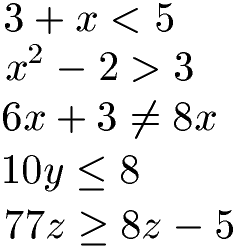Inequalities can be solved in a similar way to equations. However, there are a few points to consider. You will learn how to do this under Solving inequalities.

Systems of equations:

In some cases several equations are related. These equations must be solved together. You can recognize this by the fact that lines go over all equations to the left and right of the equations. This can look like this, for example: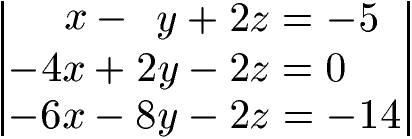Solving systems of equations usually takes a lot of work. As a rule, numerous calculation steps have to be carried out and one should not miscalculate. You can learn how to do this under solving a linear system of equations.

Show:

### Types of equations

What are the different types of equations? And how can you solve these equations? First, different types of equations are presented: First there is the quadratic equation, then the cubic equation and the biquadratic equation. The PQ formula or the ABC formula or the midnight formula can be used for the quadratic equation; the PQ formula is used in the video. For the cubic equation, the Horner scheme is applied to an example or a task. A task for the biquadratic equation is calculated last in the video. A substitution is used and presented for this purpose. I found this video on Youtube.com.

Next video »

### Solve questions with answers to equations

In this section we look at typical questions and answers about solving equations.

Q: How do I know what type of equation I have?

A: First, take a look at the powers, especially the highest exponent. In this way it can usually be seen quickly whether it is a linear equation, quadratic equation, etc. Also see if there is an equals (=) or a sign of an inequality.

Q: What topics should I look at next?

A: For example, we have this content for solving equations: